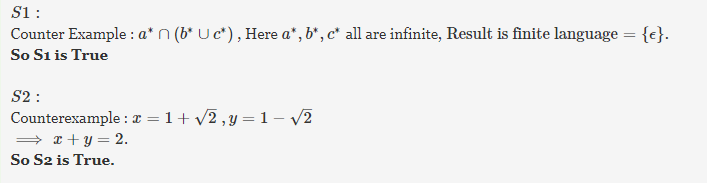# Gate CS-2001 Question Paper With Solutions

Q. 27 Consider the following statements:

```S1: There exists infinite sets A, B, C such that
A ∩ (B ∪ C) is finite.
S2: There exists two irrational numbers x and y such
that (x+y) is rational.```

Which of the following is true about S1 and S2?

(A) Only S1 is correct

(B) Only S2 is correct

(C) Both S1 and S2 are correct

(D) None of S1 and S2 is correct